# Golden Ratio Heterodynes

What is the Golden Ratio? There's two easy ways to find it. You can do it Geometrically, or Algebraically.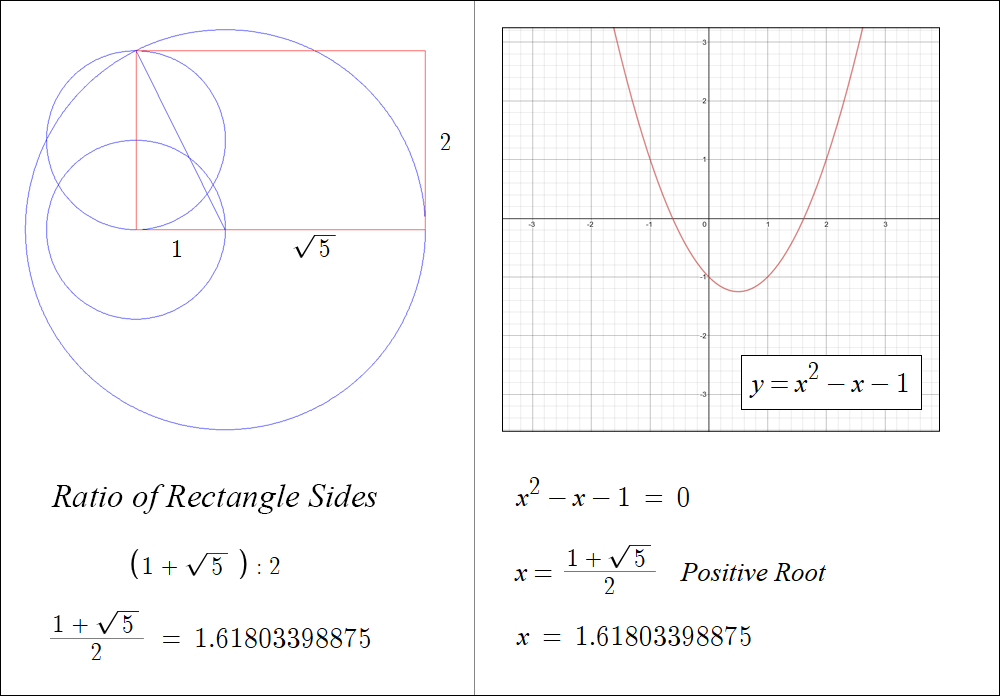The Golden Ratio has a special property: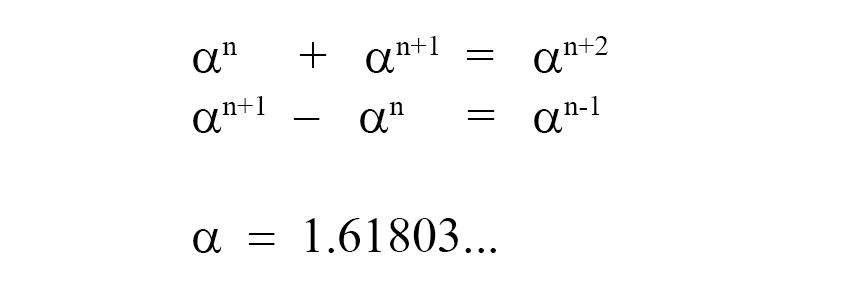Consider the Geometric Sequence:If you add together any two consecutive terms in this sequence, you get the next term. If you take the difference between any two consecutive terms, you get the previous term. This is only true when Alpha = 1.61803...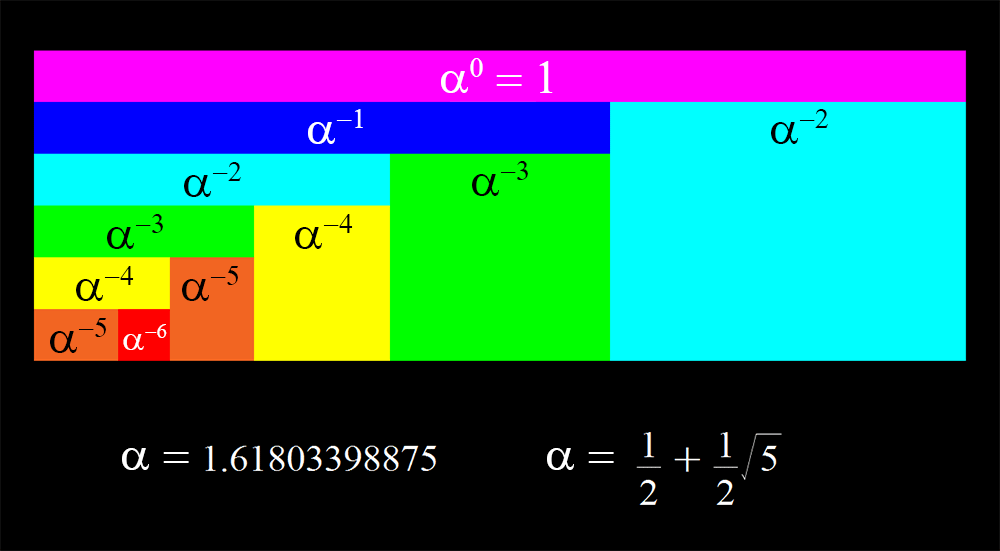Think of the terms in this sequence as the frequencies of waves.

## Heterodynes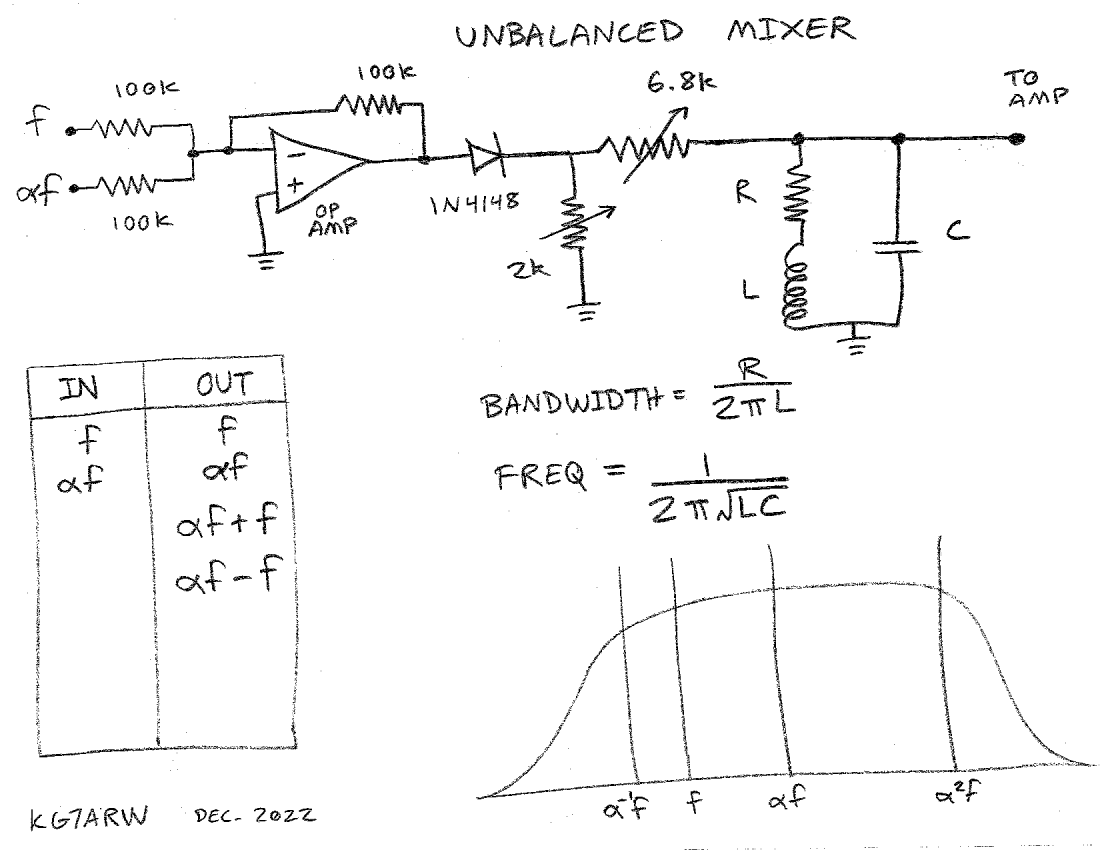Here is a simple mixer circuit. You feed in two waves: the Carrier and the Intelligence. You get out four waves: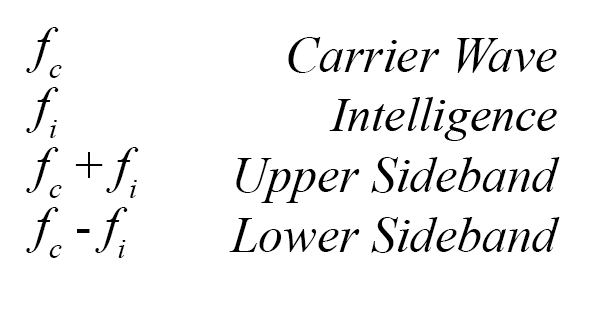The new waves created by the mixer are called Sidebands. The Upper Sideband is the sum of the two input frequencies; the Lower Sideband is the difference.

This circuit is an "Unbalanced" Mixer, which means that the Carrier and Intelligence are also present at the output, along with the Upper and Lower Sidebands. That's what we want. We want all four waves.

All it takes to heterodyne two waves is an op-amp and a diode. The op-amp sums together the input signals linearly. It is a Summing Amplifier. The diode is the non-linear element which actually does the frequency multiplication and creates the sidebands.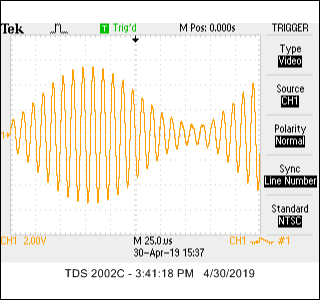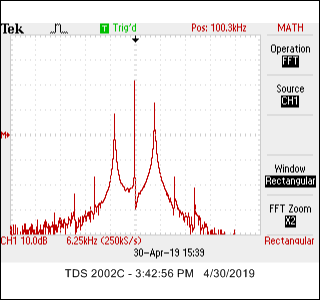Here is what a typical AM signal looks like in the Time and Frequency domains. This is a 100kHz carrier wave modulated with a 5 kHz signal. These waveforms were generated by the circuit shown above. On the FFT plot, you can see the big spike in the middle. That's the carrier at 100 kHz. There is a sideband on either side, 5kHz away. The 5kHz signal is also present, but it's off the screen.

## Golden Ratio Heterodynes

Now, let's do an experiment. Let's say our Intelligence has a frequency equal to the Carrier Wave times the Golden Ratio.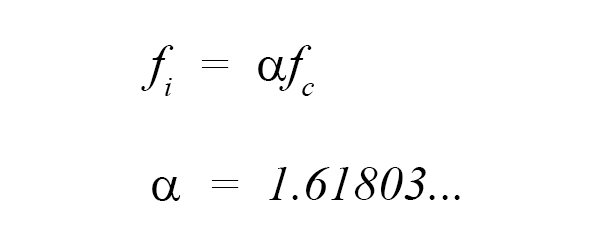If this is the case, then an interesting relationship exists between the frequencies produced by the mixer. They form a Geometric Sequence.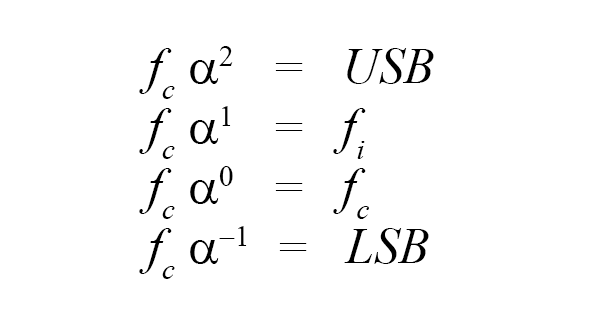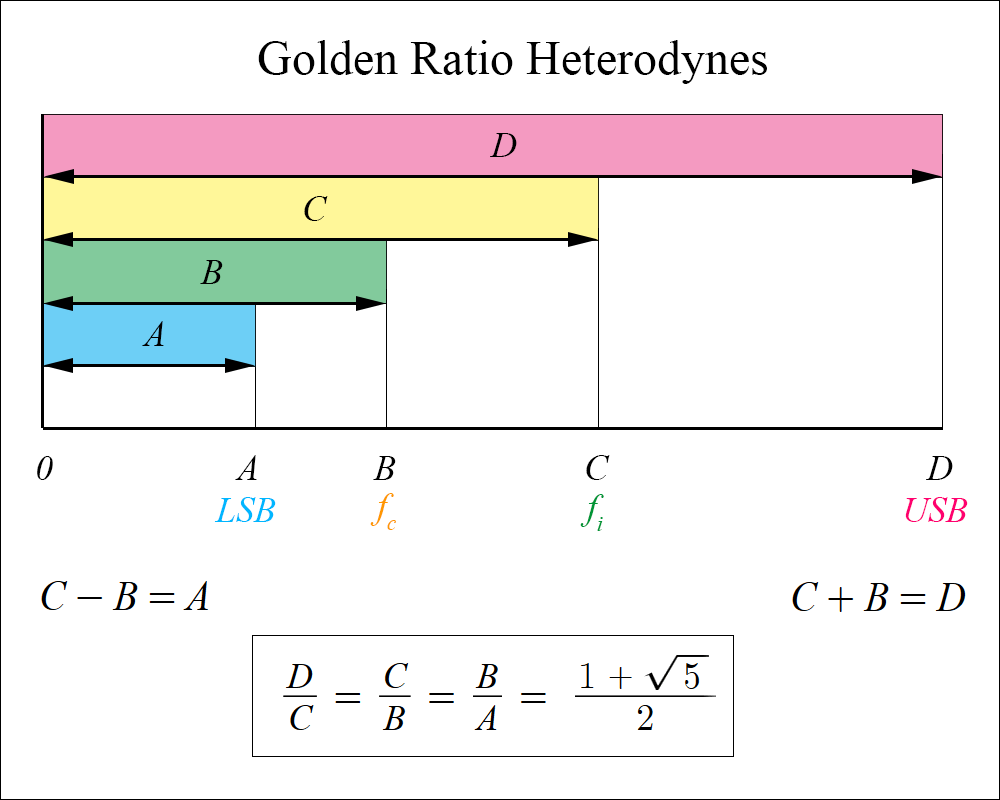We can create more terms in the sequence by feeding the output of the mixer back into the input. (Other products will also be created which are not terms in the sequence, but which bear a fractal-like relationship to it.)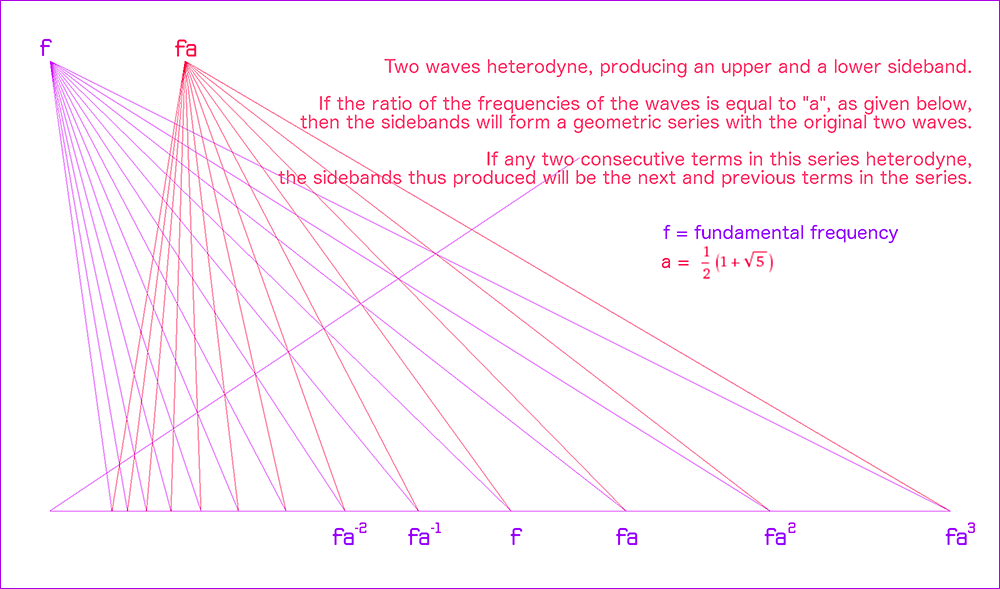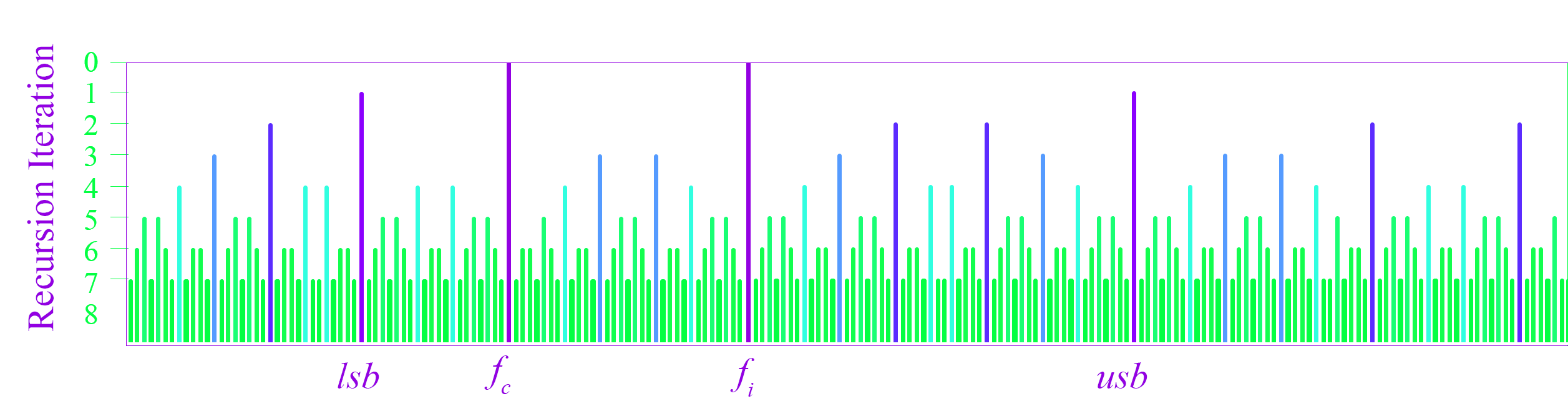Now the question naturally arises: If we think of these waves as standing waves in space, with wavelengths inversely proportional to their frequencies, what would be the appropriate geometry for a structure in which they would resonate?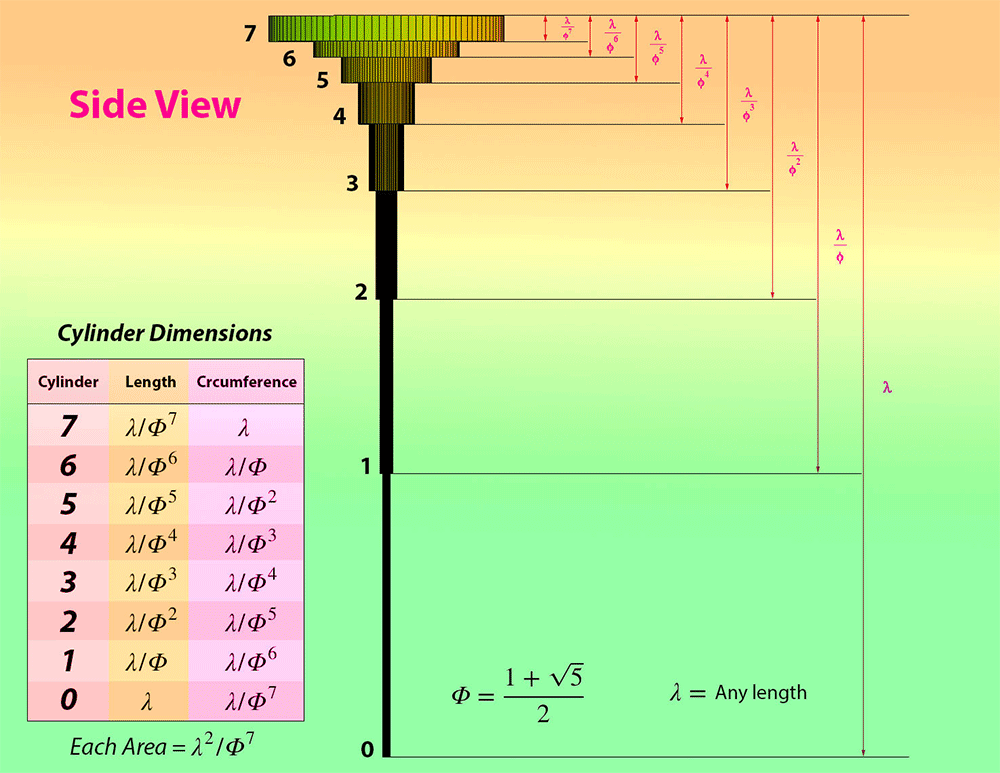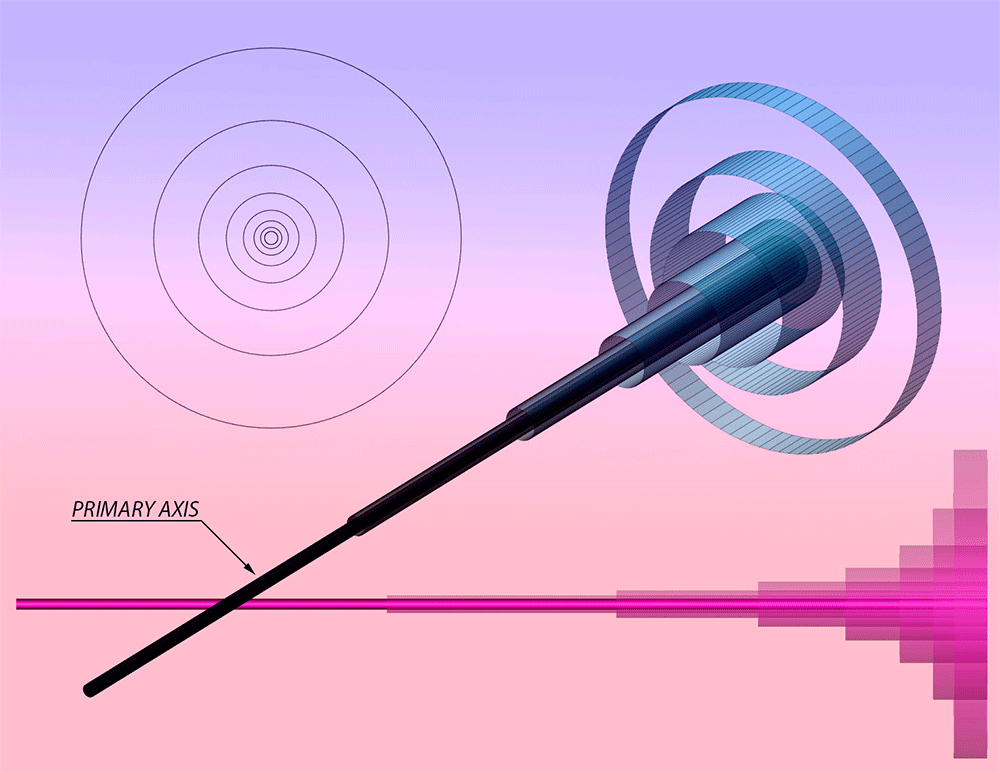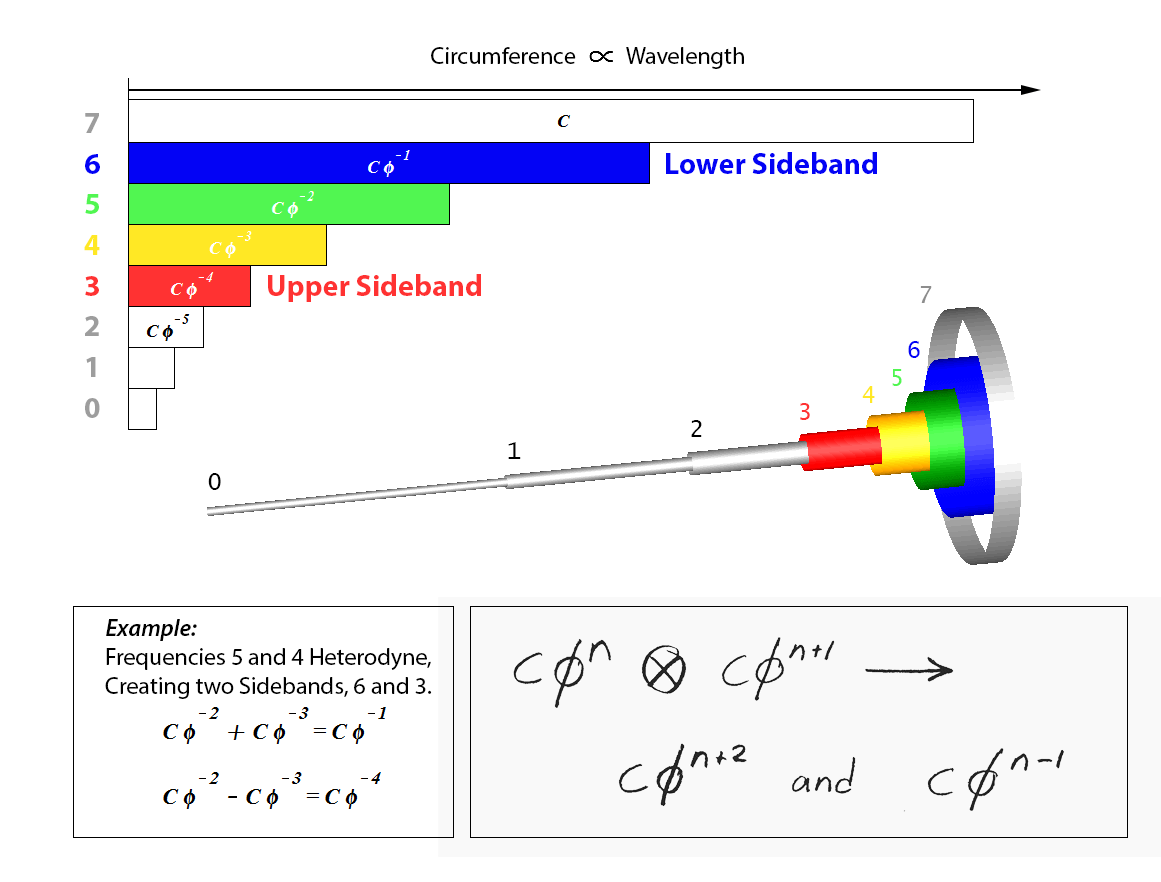The physical dimensions of this structure form a Geometric Sequence.
...to be continued...